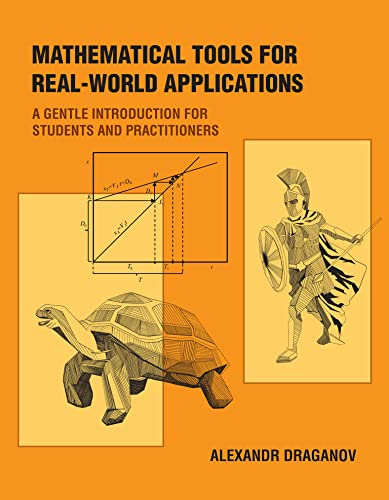# Mathematical Tools for Real-World Applications: A Gentle Introduction for Students and Practitioners

• Length: 306 pages
• Edition: 1
• Publisher:
• Publication Date: 2022-08-02
• ISBN-10: 0262543966
• ISBN-13: 9780262543965
5.0
Description

Techniques for applying mathematical concepts in the real world: six rarely taught but crucial tools for analysis, research, and problem-solving.

Many young graduates leave school with a solid knowledge of mathematical concepts but struggle to apply these concepts in practice. Real scientific and engineering problems are different from those found in textbooks: they are messier, take longer to solve, and standard solution recipes might not apply. This book fills the gap between what is taught in the typical college curriculum and what a practicing engineer or scientist needs to know. It presents six powerful tools for analysis, research, and problem-solving in the real world: dimensional analysis, limiting cases, symmetry, scaling, making order of magnitude estimates, and the method of successive approximations.

The book does not focus on formulaic manipulations of equations, but emphasizes analysis and explores connections between the equations and the application. Each chapter introduces a set of ideas and techniques and then shows how these techniques apply to a series of problems. (Knowledge of algebra and trigonometry, but not calculus, is required.) The final two chapters tie all six techniques together and apply them to two real-world problems: computing the probability of a rare, catastrophic event, and tracking a satellite with a GPS receiver. Readers will learn how to analyze, dissect, and gain insight into the results by using all the techniques presented in earlier chapters–and discover how analysis tools work on problems not concocted for a textbook. The appendix provides solutions to many of the problems found throughout the book.

Alexandr Draganov was born and raised in Kyiv, Ukraine; in light of the current war in Ukraine he will donate 100% of his royalties for the first year to support medical and humanitarian efforts there.​